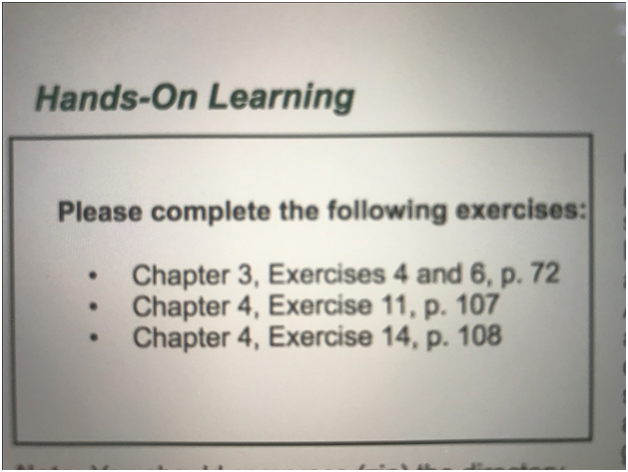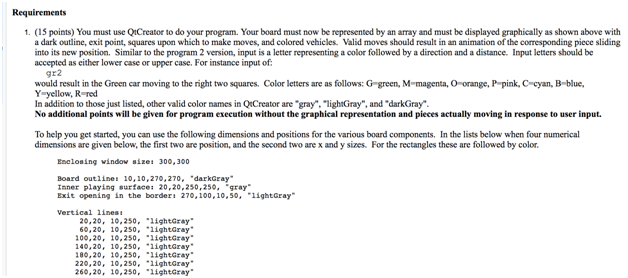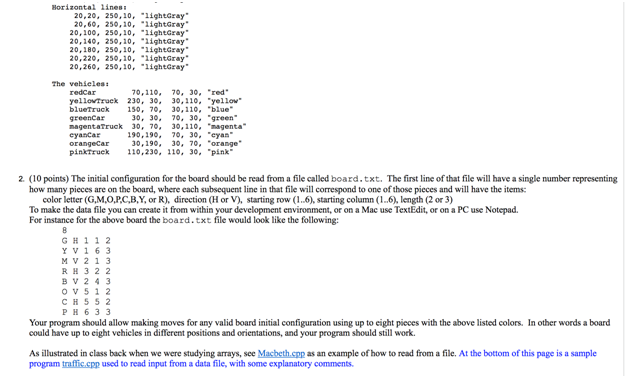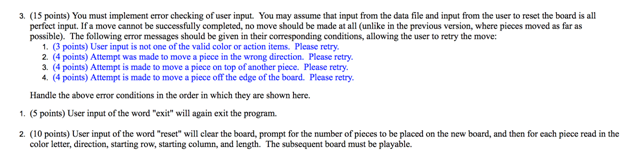# Rush hour game assignment sample

Learn how to create a rush hour game from our programming experts. We provide professional help to students who need assistance preparing this game. Reach out to us for quality academic solutions.

## Rush Hour GameTraffic.cpp

/* ——————————————————–

* Traffic.cpp

* Text-based version of the game RushHour, where the

* object is to move the vehicles such that the small

* car can exit the right of the board.

*

Running the program looks like:

Author: Dale Reed

Lab: Wed 5am

Program: #2, Traffic

Welcome to the traffic game!

Move the vehicles so that the Red car (RR) can exit

the board to the right. Each move should be of the

of the form: CDN where:

C is the vehicle to be moved

D is the direction (u=up, d=down, l=left or r=right)

N is the number of squares to move it

For example GR2 means move the G vehicle to the right

2 squares. Lower-case input such as gr2 is also

accepted. Enter x to exit the program.

– – – – – – – –

| G G . . . Y |

| P . . B . Y |

| P R RB . Y >

| P . . B . . |

| O . . . T T |

| O . F FF . |

– – – – – – – –

– – – – – – – –

| . G G . . Y |

| P . . B . Y |

| P R RB . Y >

| P . . B . . |

| O . . . T T |

| O . F FF . |

– – – – – – – –

.

.

.

*/

#include

#include // for toupper()

using namespace std;

// Global variables and constants

intNumberOfPieces = 36; // Total number of board pieces

// Variables to store the board pieces

char p1, p2, p3, p4, p5, p6,

p7, p8, p9,p10,p11,p12,

p13,p14,p15,p16,p17,p18,

p19,p20,p21,p22,p23,p24,

p25,p26,p27,p28,p29,p30,

p31,p32,p33,p34,p35,p36;

//——————————————————————–

// Display ID info

voiddisplayIDInfo()

{

printf(“Author: Dale Reed \n”);

printf(“Lab: Mon 5am \n”);

printf(“Program: #2, Traffic \n”);

printf(” \n”);

}//end displayIDInfo()

//——————————————————————–

// Display Instructions

voiddisplayInstructions()

{

cout<< “Welcome to the traffic game! ” <

<< ” ” <

<< “Move the vehicles so that the Red car (RR) can exit ” <

<< “the board to the right. Each move should be of the ” <

<< “of the form: CDN where: ” <

<< ” C is the vehicle to be moved ” <

<< ” D is the direction (u=up, d=down, l=left or r=right)” <

<< ” N is the number of squares to move it ” <

<< “For example GR2 means move the G vehicle to the right” <

<< “2 squares. Lower-case input such as gr2 is also ” <

<< “accepted. Enter ‘-‘ to reset board, or ‘x’ to exit. ” <

}//end displayInstructions()

//——————————————————————–

// Display the board, using the current values in the global variables.

voiddisplayBoard( )

{

cout<

<< “- – – – – – -” <

<< “|”<< p1<<” “<< p2 <<” “<< p3 <<” “<< p4 <<” “<< p5 <<” “<< p6 <<“|”<

<< “|”<< p7<<” “<< p8 <<” “<< p9 <<” “<< p10 <<” “<< p11 <<” “<< p12 <<“|”<

<< “|”<< p13 <<” “<< p14 <<” “<< p15 <<” “<< p16 <<” “<< p17 <<” “<< p18 <<” “<

<< “|”<< p19 <<” “<< p20 <<” “<< p21 <<” “<< p22 <<” “<< p23 <<” “<< p24 <<“|”<

<< “|”<< p25 <<” “<< p26 <<” “<< p27 <<” “<< p28 <<” “<< p29 <<” “<< p30 <<“|”<

<< “|”<< p31 <<” “<< p32 <<” “<< p33 <<” “<< p34 <<” “<< p35 <<” “<< p36 <<“|”<

<< “- – – – – – -” <

}//end displayBoard()

//——————————————————————–

// Prompt for new board characters, which should be entered as 36

// characters, such as the following, organized from easier to harder:

/*

..Y.GG

..Y…

RRY…

PPP..B

…..B

…..B

YYYL.O

..PL.O

RRP..T

..PNNT

BBB.U.

.EE.U.

RROY..

P.OY..

PRRY..

P..BBB

GGG.TK

UU..TK

GOTTPR

GO..PG

YRRE.S

YWWE.S

Y.LUUU

NNLBBB

*/

voidresetBoard()

{

cout<< “You have chosen to reset the board. ” <

<< “Enter 36 characters for the new board: “<

cin>> p1 >> p2 >> p3 >> p4 >> p5 >> p6

>> p7>> p8 >> p9 >> p10 >> p11 >> p12

>> p13 >> p14 >> p15 >> p16 >> p17 >> p18

>> p19 >> p20 >> p21 >> p22 >> p23 >> p24

>> p25 >> p26 >> p27 >> p28 >> p29 >> p30

>> p31 >> p32 >> p33 >> p34 >> p35 >> p36;

}

//——————————————————————–

// Return true if the vehicle is in more than one of the six board positions.

// If we only checked a single position we would get a false positive,

// for instance when we checked a row that overlapped a vertical vehicle.

boolisInSquares( char vehicle, // Character we’re searching for

char n1, char n2, char n3, // Six board positions

char n4, char n5, char n6)

{

intletterCount = 0;

// Count how many times the letter is found. Vehicles are at least

// two characters in length.

if( vehicle==n1) letterCount++;

if( vehicle==n2) letterCount++;

if( vehicle==n3) letterCount++;

if( vehicle==n4) letterCount++;

if( vehicle==n5) letterCount++;

if( vehicle==n6) letterCount++;

if(letterCount>= 2) {

return true; // vehicle is in the set of squares

} else {

return false; // vehicle is NOT in the set of squares

}

}//end isInSquares()

//——————————————————————–

// Swap two board characters.

void swap( char &n1, char &n2)

{

char temp = n1;

n1 = n2;

n2 = temp;

}

//——————————————————————–

// Move vehicle one square in the indicated direction. This works

// for both rows and columns, depending on which set of variables was

// sent to the function.

//

// To move right or down, start with the last character. If it is ‘.’ and

// the character to the left of it is the vehicle character, then swap

// the two characters. Continue this with the next character to the left

// until there are no more characters remaining.

//

// To move left or up, start with the first character. If it is ‘.’ and

// the character to the right of it is the vehicle character, then swap

// the two characters. Continue this with the next character to the right

// until there are no more characters remaining.

voidmoveOneSquare( char vehicle, // The character to be moved

char direction, // Direction: L, R, U or D

char&n1, char &n2, char &n3, // Six characters in the row or col

char&n4, char &n5, char &n6,

bool&thereWasAnError) // Set to true if no move was made

{

if( direction == ‘R’ || direction == ‘D’) {

if( n6==’.’ && n5==vehicle) { swap( n5, n6); thereWasAnError = false;}

if( n5==’.’ && n4==vehicle) { swap( n4, n5); thereWasAnError = false;}

if( n4==’.’ && n3==vehicle) { swap( n3, n4); thereWasAnError = false;}

if( n3==’.’ && n2==vehicle) { swap( n2, n3); thereWasAnError = false;}

if( n2==’.’ && n1==vehicle) { swap( n1, n2); thereWasAnError = false;}

}

else if( direction == ‘L’ || direction == ‘U’) {

if( n1==’.’ && n2==vehicle) { swap( n1, n2); thereWasAnError = false;}

if( n2==’.’ && n3==vehicle) { swap( n2, n3); thereWasAnError = false;}

if( n3==’.’ && n4==vehicle) { swap( n3, n4); thereWasAnError = false;}

if( n4==’.’ && n5==vehicle) { swap( n4, n5); thereWasAnError = false;}

if( n5==’.’ && n6==vehicle) { swap( n5, n6); thereWasAnError = false;}

}

}//end moveOneSquare()

//——————————————————————–

// Make the move using the direction (l,r,u,d) and distance.

// For reference, the board positions are:

// p1, p2, p3, p4, p5, p6

// p7, p8, p9, p10, p11, p12

// p13, p14, p15, p16, p17, p18

// p19, p20, p21, p22, p23, p24

// p25, p26, p27, p28, p29, p30

// p31, p32, p33, p34, p35, p36

voidmoveOneSquare( char vehicle, // Vehicle letter

char direction, // Direction of move (L,R,U,D)

bool&thereWasAnError) // Left as true if no move is made

{

// See which row or column this vehicle is in and send those board

// characters to be manipulated to make the move.

//

// First check the six rows

if(isInSquares( vehicle, p1, p2, p3, p4, p5, p6)) { // row 1

moveOneSquare( vehicle, direction, p1, p2, p3, p4, p5, p6, thereWasAnError);

}

else if( isInSquares( vehicle, p7, p8, p9, p10, p11, p12)) { // row 2

moveOneSquare( vehicle, direction, p7, p8, p9, p10, p11, p12, thereWasAnError);

}

else if( isInSquares( vehicle, p13, p14, p15, p16, p17, p18)) { // row 3

moveOneSquare( vehicle, direction, p13, p14, p15, p16, p17, p18, thereWasAnError);

}

else if( isInSquares( vehicle, p19, p20, p21, p22, p23, p24)) { // row 4

moveOneSquare( vehicle, direction, p19, p20, p21, p22, p23, p24, thereWasAnError);

}

else if( isInSquares( vehicle, p25, p26, p27, p28, p29, p30)) { // row 5

moveOneSquare( vehicle, direction, p25, p26, p27, p28, p29, p30, thereWasAnError);

}

else if( isInSquares( vehicle, p31, p32, p33, p34, p35, p36)) { // row 6

moveOneSquare( vehicle, direction, p31, p32, p33, p34, p35, p36, thereWasAnError);

}

// Now check the six columns

else if( isInSquares( vehicle, p1, p7, p13, p19, p25, p31)) { // column 1

moveOneSquare( vehicle, direction, p1, p7, p13, p19, p25, p31, thereWasAnError);

}

else if( isInSquares( vehicle, p2, p8, p14, p20, p26, p32)) { // column 2

moveOneSquare( vehicle, direction, p2, p8, p14, p20, p26, p32, thereWasAnError);

}

else if( isInSquares( vehicle, p3, p9, p15, p21, p27, p33)) { // column 3

moveOneSquare( vehicle, direction, p3, p9, p15, p21, p27, p33, thereWasAnError);

}

else if( isInSquares( vehicle, p4, p10, p16, p22, p28, p34)) { // column 4

moveOneSquare( vehicle, direction, p4, p10, p16, p22, p28, p34, thereWasAnError);

}

else if( isInSquares( vehicle, p5, p11, p17, p23, p29, p35)) { // column 5

moveOneSquare( vehicle, direction, p5, p11, p17, p23, p29, p35, thereWasAnError);

}

else if( isInSquares( vehicle, p6, p12, p18, p24, p30, p36)) { // column 6

moveOneSquare( vehicle, direction, p6, p12, p18, p24, p30, p36, thereWasAnError);

}

}//end makeMove()

//——————————————————————–

// We are done when p18, the square adjacent to the exit position, has

// ‘R’ in it, so the red car can exit.

bool done()

{

return p18==’R’; // true if p18 equals ‘R’, false otherwise

}

//——————————————————————–

/* Board and corresponding global variable values are:

– – – – – – – –

1 | G G . . . Y |

7 | P . . B . Y |

13 | P R RB . Y >

19 | P . . B . . |

25 | O . . . T T |

31 | O . F FF . |

– – – – – – – –

*/

int main()

{

char vehicle; // Extracted from user input

char direction; // Extracted from user input (u,d,l,r)

int distance; // Extracted from user input

intmoveNumber = 1; // Counts and displays number of moves

boolthereWasAnError = false; // Gets set if a move attempts to move

// out of bounds or over another piece.

displayIDInfo(); // Display ID info

displayInstructions(); // Display game instructions

// Set the board values

p1=’G’; p2=’G’; p3=’.’; p4=’.’; p5=’.’; p6=’Y’;

p7=’P’; p8=’.’; p9=’.’;p10=’B’;p11=’.’;p12=’Y’;

p13=’P’;p14=’R’;p15=’R’;p16=’B’;p17=’.’;p18=’Y’;

p19=’P’;p20=’.’;p21=’.’;p22=’B’;p23=’.’;p24=’.’;

p25=’O’;p26=’.’;p27=’.’;p28=’.’;p29=’T’;p30=’T’;

p31=’O’;p32=’.’;p33=’F’;p34=’F’;p35=’F’;p36=’.’;

// Display the initial board

displayBoard();

// Main loop to play program

do {

// Prompt for and get user input, including checking for ‘x’ to exit

cout< “;

cin>> vehicle;

vehicle = toupper( vehicle); // convert to upper case to simplify comparisons

if( vehicle == ‘X’) {

break; // break out of main playing loop to exit the game

}

else if( vehicle == ‘-‘) {

// Reset the board and restart loop

resetBoard();

moveNumber = 1;

vehicle = ‘ ‘;

displayBoard();

continue;

}

// Get the direction (‘l’,’r’,’u’,’d’) and distance (1..4)

cin>> direction >> distance;

// Convert direction to upper case to simplify future comparisons

direction = toupper( direction);

// Make the move. Move the whole vehicle one square, repeating this for the

// total number of squares that it is supposed to be moved.

for(int i=0; i

thereWasAnError = true; // Error flag gets reset to false when a valid move is made

moveOneSquare( vehicle, direction, thereWasAnError);

if(thereWasAnError) {

// No move was made and error flag was left as true

cout<< “*** Move was attempted, but couldn’t be completed. *** ” <

break; // Stop attempting to make any further moves, so error message

// is only shown once.

}

}

// Display the new board reflecting the recent move

displayBoard();

moveNumber++; // Increment the move number

}while( !done()); // Red car ‘R’ at this position is the exit point for a win

// Display appropriate ending message

if( done() ) {

cout<

}

else {

cout<< “Better luck next time. ” <

}

cout<

cout<< “Thank you for playing. Exiting…” <

return 0;

}//end main()

traffic-2.cpp

// Graphics demo program for getting started with QtCreator

//

#include

#include “gobjects.h” // for all graphics objects

#include // For file input

using namespace std;

int main() {

ifstreaminputFileStream; // declare the input file stream

charuserInput;

double x1,y1,x2,y2,x3,y3;

// open input file and verify.

// You must first:

// 1. Outside of QtCreator go into your project’s resources folder, copy readme.txt into data.txt

// 2. Edit data.txt to have the datafile information as shown on the assignment page

// 3. Now WITHIN QtCreator right-click on the “res” folder (under “Other files”) and

// choose “add new” and add the new data.txt file so it shows up in your environment.

inputFileStream.open(“data.txt”);

if( !inputFileStream.is_open()) {

cout<< “Could not find input file data.txt. Exiting…” <

exit( -1);

}

inthowManyVehicles;

inputFileStream>>howManyVehicles;

cout<< “Number of vehicles is: ” <

char vehicle, direction;

intxPosition, yPosition, length;

for(int i=0; i

inputFileStream>> vehicle >> direction >>xPosition>>yPosition>> length;

cout<< “This vehicle is: ” << vehicle << ” ” << direction << ” ”

<< ” ” <

}

cout<< “Welcome to CS 141” <

cout<< “This program draws a rectangle, triangle and circle.\n”

<< “Press a/d to move the triangle left/right, or x to exit.”<

// Create a graphics window, which will be used to draw graphical shapes

GWindowgraphicsWindow(400, 400); // Set the size

graphicsWindow.setWindowTitle(“CS 141 Lab Sample Drawing”);

// Create a rectangle

GRect *rectangle = new GRect(0, 0, 200, 100);

rectangle->setFilled(true);

rectangle->setColor(“RED”);

// Add it to the graphics window. Without this it will exist, but will not show up.

// Create a polygon, in this case with three sides, so it is a triangle

GPolygon *triangle = new GPolygon();

x1 = 100; y1 = 100;

x2 = 100; y2 = 200;

x3 = 50; y3 = 100;

triangle->setFilled(true);

triangle->setColor(“BLUE”);

// Add it to the graphics window. Without this it will exist, but will not show up.

// Create an oval

GOval *circle = new GOval(200, 200, 60, 60);

circle->setFilled(true);

circle->setColor(“GREEN”);

// Add it to the graphics window. Without this it will exist, but will not show up.

// Create a label

GLabel *message = new GLabel(“Welcome!”, 200,200);

// Add it to the graphics window. Without this it will exist, but will not show up.

// Make it the current window, but only for one second

graphicsWindow.requestFocus();

pause(1000); // time in milliseconds

// Move the triangle, depending on user input

while(true){

cin>>userInput;

userInput = toupper(userInput);

// Make triangle move

if(userInput == ‘A’) {

triangle->move(-5,0); // decrement the x values for the triangle, moving to the left

}

else if( userInput == ‘D’) {

triangle->move(5,0); // increment the x values for the triangle, moving to the right

}

else if( userInput == ‘X’) {

break; // exit the program

}

}

// Close the windows, first the graphics window, then the console window.

graphicsWindow.requestFocus();

graphicsWindow.close();

exitGraphics();

return 0;

Solution

main.cpp

// Graphics demo program for getting started with QtCreator

//

#include

#include “gobjects.h” // for all graphics objects

#include “gevents.h”

#include

#include

using namespace std;

classMoveBlock

{

boolHorV;

intstRow;

intstColumn;

int length;

string color;

GRect *block;

public:

MoveBlock(boolHorV,intstRow,intstColumn,intlength,string color)

{

this->HorV=HorV;

this->stRow=stRow;

this->stColumn=stColumn;

this->color=color;

this->length=length;

if(!HorV)

{

block= new GRect(23+(360/6)*stRow,23+(360/6)*stColumn ,360/6-20 ,(360/6)*length-20 );

}

else

{

block= new GRect(23+(360/6)*stRow,23+(360/6)*stColumn ,(360/6)*length-20,360/6-20 );

}

block->setFilled(true);

block->setColor(color);

}

void move(double distX,doubledistY)

{

if(!HorV)

{

if(block->getY()+distY+(360/6)*(length) <380&&block->getY()+distY>15)

block->move(0,distY);

}

else

{

if(block->getX()+(360/6)*(length)+distX<380&&block->getX()+distX>15)

block->move(distX,0);

}

}

GRect *getBlock() const;

intgetStRow() const;

intgetStColumn() const;

intgetLength() const;

voidsetLength(int value);

boolgetHorV() const;

};

int main() {

constint size =6;

vectorAllBlocks;

char* temp;

booltempH;

inttempCol=0;

inttempRow=0;

inttempSize=0;

intcountSize;

std::ifstreammyfile(“board.txt”);

if (myfile.is_open())

{

char c;

myfile>>countSize;

for(int i=0;i

c = myfile.get();

c = myfile.get();

std::cout<< c;

switch(c)

{

case ‘G’:

{

temp=”green”;

break;

}

case ‘M’:

{

temp=”magenta”;

break;

}

case ‘O’:

{

temp=”orange”;

break;

}

case ‘P’:

{

temp=”pink”;

break;

}

case ‘C’:

{

temp=”cyan”;

break;

}

case ‘B’:

{

temp=”blue”;

break;

}

case ‘Y’:

{

temp=”yellow”;

break;

}

case ‘R’:

{

temp=”red”;

break;

}

default:

{

return 0;

}

}

c = myfile.get();

c = myfile.get();

if(c==’H’)

{

tempH=true;

}

else

{

tempH=false;

}

c = myfile.get();

c = myfile.get();

tempRow=c-‘0’;

c = myfile.get();

c = myfile.get();

tempCol=c-‘0’;

c = myfile.get();

c = myfile.get();

tempSize=c-‘0’;

AllBlocks.push_back(new MoveBlock(tempH,tempCol-1,tempRow-1,tempSize,temp));

}

}

else

{

cout<<“ba d file name board.txt is need”<

}

// Create a graphics window, which will be used to draw graphical shapes

GWindowgraphicsWindow(400, 400); // Set the size

graphicsWindow.setWindowTitle(“CS 141 Lab Sample Drawing”);

// Create a rectangle

GRect *background1 = new GRect(0, 0, 400, 400);

background1->setFilled(true);

background1->setColor(“darkgray”);

// Add it to the graphics window. Without this it will exist, but will not show up.

GRect *background2 = new GRect(15, 15, 370, 370);

background2->setFilled(true);

background2->setColor(“gray”);

// Add it to the graphics window. Without this it will exist, but will not show up.

for(int i=0;i

{

for(int j=0;j

{

GRect *block = new GRect(25+(360/size)*i,25+(360/size)*j ,360/size-25 ,360/size-25 );

block->setFilled(true);

block->setColor(“darkgray”);

}

}//DARK GRAY BLOCKS

GRect *finish = new GRect(380,25+(360/6)*2 ,360/6-15 ,360/6-15 );

finish->setFilled(true);

finish->setColor(“gray”);

for(int i=0;i

// Make it the current window, but only for one second

graphicsWindow.requestFocus();

pause(100); // time in milliseconds

boolisMove=false;

GRect* curBl=0;

GRect* tempBl=0;

MoveBlock *curMove=0;

// Move the triangle, depending on user input

while (true) {

doublestartX;

doublestartY;

GMouseEvent e = waitForEvent();

if(e.getEventType() == MOUSE_CLICKED)

{

if(!isMove)

{

cout<< “click” <

startX = e.getX();

startY = e.getY();

cout<<“x ;”<

for(int j=0;j

{

curBl=AllBlocks[j]->getBlock();

if(curBl->getX()getX()+curBl->getWidth()>startX)

{

if(curBl->getY()getY()+curBl->getHeight()>startY)

{

curMove=AllBlocks[j];

}

}

}

isMove=true;

}

else

{

isMove=false;

}

}

else if (e.getEventType() == MOUSE_MOVED) {

if(isMove)

{

double x = e.getX()- startX;

double y = e.getY()- startY;

boolisMove=true;

if(!curMove->getHorV())

{

for(int j=0;j

{

if(AllBlocks[j]==curMove)continue;

tempBl=AllBlocks[j]->getBlock();

if(curBl->getY()>tempBl->getY()+tempBl->getHeight())

{

if(tempBl->getX()getX()&&tempBl->getX()+tempBl->getWidth()>curBl->getX() )

{

if(tempBl->getX()getX()+curBl->getWidth()&& tempBl->getX()+tempBl->getWidth()>curBl->getX()+curBl->getWidth() )

{

isMove=false;

continue;

}

}

}

}

}

else

{

for(int j=0;j

{

if(AllBlocks[j]==curMove)continue;

tempBl=AllBlocks[j]->getBlock();

if (curBl->getX()>tempBl->getX()+tempBl->getWidth()) {

if(tempBl->getY()getY()&&tempBl->getY()+tempBl->getHeight()>curBl->getY() )

{

if(tempBl->getY()getY()+curBl->getHeight()&& tempBl->getY()+tempBl->getHeight()>curBl->getY()+curBl->getHeight() )

{

isMove=false;

continue;

}

}

}

}

}

if(isMove)

curMove->move(x,y);

}

}

}

// Close the windows, first the graphics window, then the console window.

graphicsWindow.requestFocus();

graphicsWindow.close();

exitGraphics();

return 0;

}

intMoveBlock::getStRow() const

{

returnstRow;

}

intMoveBlock::getStColumn() const

{

returnstColumn;

}

intMoveBlock::getLength() const

{

return length;

}

voidMoveBlock::setLength(int value)

{

length = value;

}

boolMoveBlock::getHorV() const

{

returnHorV;

}

GRect *MoveBlock::getBlock() const

{

return block;

}